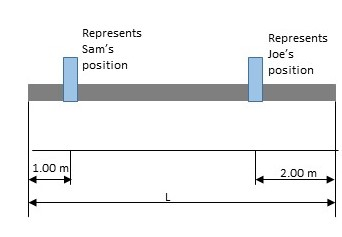# A uniform beam of length L= 7.10 m and weight 3.80x10^2 N is carried by two workers, Sam and Joe,...

## Question:

A uniform beam of length L= 7.10 m and weight 3.80x{eq}10^2 {/eq} N is carried by two workers, Sam and Joe, as shown in the figure below. Determine the force that each person exerts on the beam.## Equilibrium

In a state of equilibrium, there are no net forces on the system. This means the sum of all forces must be zero. This applies to both linear and rotational forces.

## Answer and Explanation:

The beam is at equilibrium, so the the net force, and the net torque, must both be zero. And since the beam is held horizontally, and is uniform, there is no torque due to it's weight distribution.

{eq}F_N=F_S+F_J+F_g=0 {/eq}

{eq}\tau_N=\tau_S+\tau_J=0 {/eq}

Since the beam is uniform, it's center of mass is at it's geometric center. To determine the torque exerted by Sam and Joe, we need to find their radii from the center of mass. This will be their distances from their closer edges subtracted from the distance from either edge to the center.

{eq}r_S=\frac{L}{2}-d_S=\frac{7.10m}{2}-1.00 m=2.55 m {/eq}

{eq}r_J=\frac{L}{2}-d_J=\frac{7.10 m }{2} - 2 m = 1.55 m {/eq}

Assuming Sam and Joe both lift straight upwards, their force is perpendicular to the radius of the horizontal beam. This reduces their torques to simply the product of their forces and their radii. Since we known their on opposite ends of the center of mass, and both lifting upwards, one of their torques will be negative. By convention, we'll say Sam's torque is negative since it is clockwise.

{eq}\tau_S=-F_Sr_S {/eq}

{eq}\tau_J=F_Jr_J {/eq}

Plugging these into the torque equilibrium expression, we have:

{eq}-F_Sr_S+F_Jr_J=0 {/eq}

{eq}F_Sr_S=F_Jr_J {/eq}

We can now turn this into an expression for one force in terms of the other.

{eq}F_S=F_J \frac{r_J}{r_S} {/eq}

Now plug this into the force equilibrium expression.

{eq}F_S+F_J+F_g=0 {/eq}

{eq}F_J \frac{r_J}{r_S}+F_J+F_g=0 {/eq}

{eq}F_J(1+ \frac{r_J}{r_S})+F_g=0 {/eq}

Rearrange to solve for the force Joe exerts.

{eq}F_J=-\frac{F_g}{1+ \frac{r_J}{r_S}} {/eq}

We can solve this analytically with the given information. Note that the force exerted by Joe is in the opposite direction of gravity, so we'll call gravity negative and upward positive.

{eq}F_J=\frac{380 N}{1+ \frac{1.55 m }{2.55}}=236 N {/eq}

Plug this back into the expression for Sam's force in terms of Joe's.

{eq}F_S=F_J \frac{r_J}{r_S} {/eq}

{eq}F_S=(236N) \frac{1.55m}{2.55m}=144 N {/eq}

#### Learn more about this topic:Equilibrium: Translational & Rotational

from UExcel Physics: Study Guide & Test Prep

Chapter 8 / Lesson 1
56K